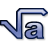A view into the mind of Jason

Welcome to Evilness
Friday, September 29 2023 @ 05:23 MDT

# F stops between f numbersFor the number of f stops between two f numbers (e.g. f11.8 and 7.4). Given f_1 and f_2:

i=log(f_1/f_2) / log(sqrt(2))

where i is the difference in f stops for exposure.

So for f11.8 and 7.4:

i=log(11.8/7.4)/(log(sqrt(2)) = 1.35 f stops.

This is 2^i times the amount of light (in this example 2^1.35=2.55 times the amount of light at f7.4 than at 11.8).

## Story Options

F stops between f numbers | 0 comments | Create New Account